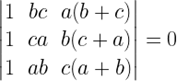NCERT Solutions for Maths Class 12 Exercise 4.2

# NCERT Solutions for Maths Class 12 Exercise 4.2

## NCERT Solutions for Maths Class 12 Exercise 4.2

Using the property of determinants and without expanding in Questions 1 to 5, prove that:

### Maths Class 12 Ex 4.2 Question 1.

Solution:

[Here, in the first determinant, C1 = C3 and in the second determinant, C2 = C3. Therefore, the value of each of the determinants is equal to zero.]
= 0 + 0
= 0
= R.H.S.

Solution:

### Maths Class 12 Ex 4.2 Question 4.Solution:

[Since, C1 and C3 are proportional, therefore the value of this determinant is 0.]

= 0

= R.H.S.

### Maths Class 12 Ex 4.2 Question 5.

Solution:

By using properties of determinants in Questions 6 to 14, show that:

### Maths Class 12 Ex 4.2 Question 15.

Let A be a square matrix of order 3 × 3, then |kA| is equal to
(A) k|A|                   (B) k²|A|                 (C) k³|A|                 (D) 3k|A|

Solution:

Hence, option (C) is correct.

### Maths Class 12 Ex 4.2 Question 16.

Which of the following is correct:
(A) Determinant is a square matrix.
(B) Determinant is a number associated to a matrix.
(C) Determinant is a number associated to a square matrix.
(D) None of these.

Solution:
We know that to every square matrix, A = [aij] of order n, we can associate a number called the determinant of square matrix A, where aij = (i, j)th element of A.

Thus, the determinant is a number associated to a square matrix.

Hence, option (C) is correct.